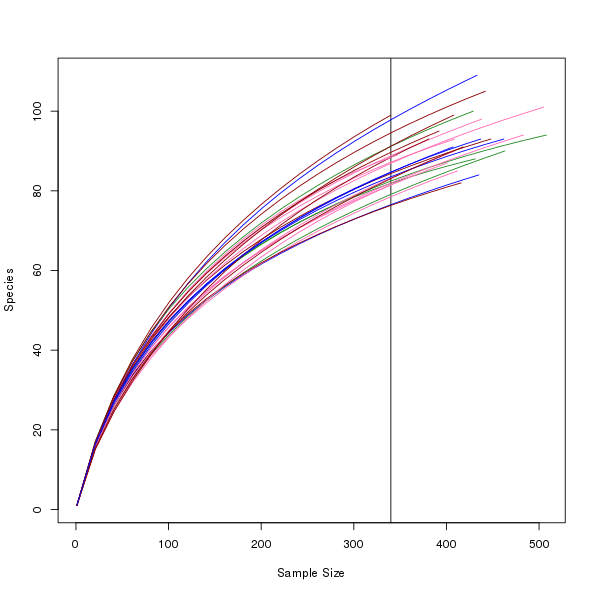[This article was first published on From the Bottom of the Heap - R, and kindly contributed to R-bloggers]. (You can report issue about the content on this page here)
Want to share your content on R-bloggers? click here if you have a blog, or here if you don't.

I was sent an email this week by a vegan user who wanted to draw rarefaction curves using `rarecurve()` but with different colours for each curve. The solution to this one is quite easy as `rarecurve()` has argument `col` so the user could supply the appropriate vector of colours to use when plotting. However, they wanted to distinguish all 26 of their samples, which is certainly stretching the limits of perception if we only used colour. Instead we can vary other parameters of the plotted curves to help with identifying individual samples.

To illustrate, I’ll use the Barro Colorado Island data set `BIC` that comes with vegan. I just take the first 26 samples as this was the data set size my correspondent indicated they had available.

```library("vegan")

This is vegan 2.2-1

data(BCI, package = "vegan")
BCI2 <- BCI[1:26, ]
raremax <- min(rowSums(BCI2))
raremax

 340
```

`raremax` is the minimum sample count achieved over the 26 samples. We will rarefy the sample counts to this value.

To set up the parameters we might use for plotting, `expand.grid()` is a useful helper function

```col <- c("black", "darkred", "forestgreen", "orange", "blue", "yellow", "hotpink")
lty <- c("solid", "dashed", "longdash", "dotdash")
pars <- expand.grid(col = col, lty = lty, stringsAsFactors = FALSE)

col   lty
1       black solid
2     darkred solid
3 forestgreen solid
4      orange solid
5        blue solid
6      yellow solid
```

Then we can call `rarecurve()` as follows with the new graphical parameters

```out <- with(pars[1:26, ],
rarecurve(BCI2, step = 20, sample = raremax, col = col,
lty = lty, label = FALSE))
```

Note that I saved the output from `rarecurve()` in object `out`. This object contains everything we need to draw our own version of the plot if we wish. For example, we could use fewer colours and alter the line thickness1 instead to make up the required number of combinations.

```col <- c("black", "darkred", "forestgreen", "hotpink", "blue")
lty <- c("solid", "dashed", "dotdash")
lwd <- c(1, 2)
pars <- expand.grid(col = col, lty = lty, lwd = lwd,
stringsAsFactors = FALSE)

col    lty lwd
1       black  solid   1
2     darkred  solid   1
3 forestgreen  solid   1
4     hotpink  solid   1
5        blue  solid   1
6       black dashed   1
```

Using the information in `out` returned by `rarecurve()` we can get almost the same plot using the following code to draw the elements by hand

```Nmax <- sapply(out, function(x) max(attr(x, "Subsample")))
Smax <- sapply(out, max)
plot(c(1, max(Nmax)), c(1, max(Smax)), xlab = "Sample Size",
ylab = "Species", type = "n")
abline(v = raremax)
for (i in seq_along(out)) {
N <- attr(out[[i]], "Subsample")
with(pars, lines(N, out[[i]], col = col[i], lty = lty[i], lwd = lwd[i]))
}
```

Having done this, I don’t believe this is a useful graphic because we’re trying to distinguish between too many samples using graphical parameters. Where I do think this sort of approach might work is if the samples in the data set come from a few different groups and we want to colour the curves by group.

```col <- c("darkred", "forestgreen", "hotpink", "blue")
set.seed(3)
grp <- factor(sample(seq_along(col), nrow(BCI2), replace = TRUE))
cols <- col[grp]
```

The code above creates a grouping factor `grp` for illustration purposes; in real analyses you’d have this already as a factor variable in you data somewhere. We also have to expand the `col` vector because we are plotting each line in a loop. The plot code, reusing elements from the previous plot, is shown below:

```plot(c(1, max(Nmax)), c(1, max(Smax)), xlab = "Sample Size",
ylab = "Species", type = "n")
abline(v = raremax)
for (i in seq_along(out)) {
N <- attr(out[[i]], "Subsample")
lines(N, out[[i]], col = cols[i])
}
```An attempt at rarefaction curves output with custom colours per groups of curves.

1. We can’t use the approach outlined in this example to vary `lwd` because of the way `rarecurve()` draws the individual curves, in a loop. We have no way to tell `rarecurve()` to use the ith element of a vector of `lwd` values.